## Trigonometry Values Pi/11

Trigonometric functions offoran integer cannot be expressed in terms of sums, products, and finite root extractions on real rational numbers because 11 is not a Fermat Prime. This also means that the Undecagon is not a Constructible Polygon.

However, exact expressions involving roots of complex numbers can still be derived using the trigonometric identity(1)
Using the identities from Beyer (1987, p. 139),(2)(3)

gives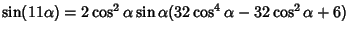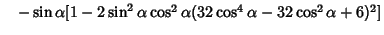(4)
Now, letand, then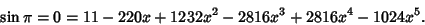(5)

This equation is an irreducible Quintic Equation, so an analytic solution involving Finite Root extractions does not exist. The numerical Roots are, 0.29229, 0.57115, 0.82743, 0.97974. So,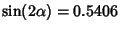,,,. From one of Newton's Identities,(6)(7)(8)
The trigonometric functions of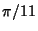also obey the identity(9)

Beyer, W. H. Trigonometry.'' CRC Standard Mathematical Tables, 28th ed. Boca Raton, FL: CRC Press, 1987.